Courses

# Surveying (Part - 9) Civil Engineering (CE) Notes | EduRev

## Civil Engineering (CE) : Surveying (Part - 9) Civil Engineering (CE) Notes | EduRev

The document Surveying (Part - 9) Civil Engineering (CE) Notes | EduRev is a part of the Civil Engineering (CE) Course Civil Engineering SSC JE (Technical).
All you need of Civil Engineering (CE) at this link: Civil Engineering (CE)

Surveying (Part 9)

SIMPLE CURVES

Curve :  A regular curved path followed by a railway by a railway or highway alignment is called a curve.A curve may be either  circular, parabolic or spiral and is always tangential to the two straight directions at its ends.

Types of Curve:

1. Simple curve : This is a type of circular curve
2. Compound curve: This, is a type of circular curve
3. Reverse curve: This is a type of circular curve
4. Transition curve

1. SIMPLE CURVE : The curve which is a single arc of a circle is known as simple curve or a simple circular curve. It is tangential to both the straights ATt and CT2.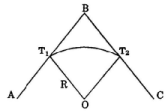2. COMPONOUND CURVE : A curve which consists of two or more arce of different circles with different radii having different centres lying on the same side of the common tangent is known as compound curve .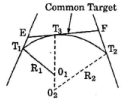3. REVERSE CURVE : A curve which consists of two area of different circles of same or different radii, is known as a reverse curve. In such curves the centre areas are on the opposite sides of the curve. The two areas turn in opposite directions with a common tangent at the junction.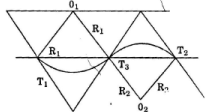4. Transition Curve : A non - circular curve introduced between a straight and a circular curve is known as a transition curve

NOTATION FOR CIRCULAR CURVE :
TANGENTS : The straight line AB and BC, which are connected by the curve are called the tangents or straights to the curve.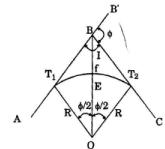Point of intersection or vertex : The point (B) at which the two tangent lines AB and 5 7 intersect is known as the point of intersection (P.I.) or the vertex.

Right and Left Hand Curve: If the curve deflects to the right of the direction of the progress of survey (AB), is called a right-hand curve, if to the left, it is called a left hand curve.

Back Tangents : The tangent AB at T1 the point of commencement of the curve, is called back tangent’ or first tangent.
Forward Tangent: The tangent BE at T2 the end point of the curve is called forward taugent or second tangent.

Angle of Deflection : The angle through which forward tangent deflects, is called angle of deflection of the curve. It may be either to the right or to the left.

Tangent Distance : The distance from the point of intersection to the tangent point (T.P) is called the tangent distance or Tangent length (BT1 and BT2).

Long Chord: The chord joining the point of curve and the point of tangency is called long chord.

Length of the Curve : The total length of the curve from the tangent curve to the curve tangent or the total length of the curve from tengent point to point of tengeny is called length of the curve.

MID Ordinate: The ordinate joining the mid point of the curve and long chord, is called mid- ordinate it is also known as versed sign.

Normal Chord: A chord between two successive regular pegs on the curve, is called a normal chord.

Sub-Chord : When a chord is shorter than the normal chord, it is called a sub-chord. These sub-chords generally occur at the beginning and at the end of the curve.
Elements of the Simple Curve : Tengent length = BT1 = BT2 = ?
In DOBT,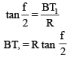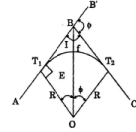Tengent length BT1 = BT2 = R tan (f/2)........... (i)
Length of the long chord = T1T2 = 2T1E = 2R sin(f/2) ............... (ii)
length of the curve, (l) = f ×R (in radian) =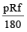.............. (iii)
Apex distance = BF = OB - OF where, cos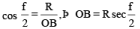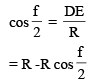Versed sine of the curve = EF = OF, OE ,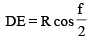..........................(iv)
= R -R cos  (f/2)

DE = R cos (f/2)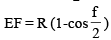Degree of Curve : Degree of curve may be defined either with respect to a fixed length or an are of the curve or with respect to a fixed length of a normal chord of the curve.

Fixed length of an are : The degree of curve may be defined as the central angle of the curve that is subtended by an arc of 30 metres (or 100 ft). It is adopted for railway curves.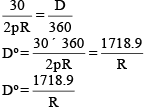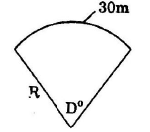Based on Fixed Length of a Chord : The degree of a cure may be defined a fixed central angle of the curve that is subtended by a chord of 30 metres lengthl.It is adopted for road curve.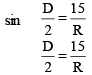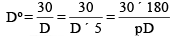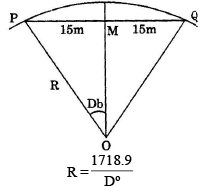Offer running on EduRev: Apply code STAYHOME200 to get INR 200 off on our premium plan EduRev Infinity!

## Civil Engineering SSC JE (Technical)

109 docs|50 tests

,

,

,

,

,

,

,

,

,

,

,

,

,

,

,

,

,

,

,

,

,

;